# CHAPTER 8 SAMPLING DISTRIBUTIONS Outline Central limit theorem

• Slides: 11CHAPTER 8 SAMPLING DISTRIBUTIONS Outline • Central limit theorem • Sampling distribution of the sample mean 1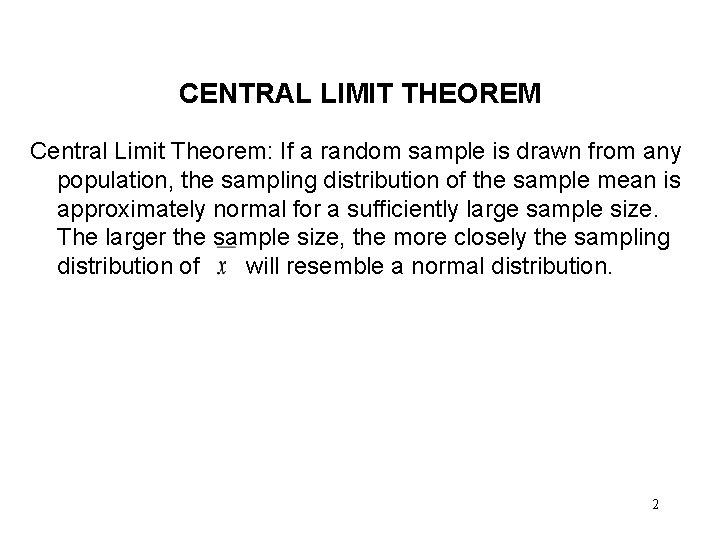CENTRAL LIMIT THEOREM Central Limit Theorem: If a random sample is drawn from any population, the sampling distribution of the sample mean is approximately normal for a sufficiently large sample size. The larger the sample size, the more closely the sampling distribution of will resemble a normal distribution. 2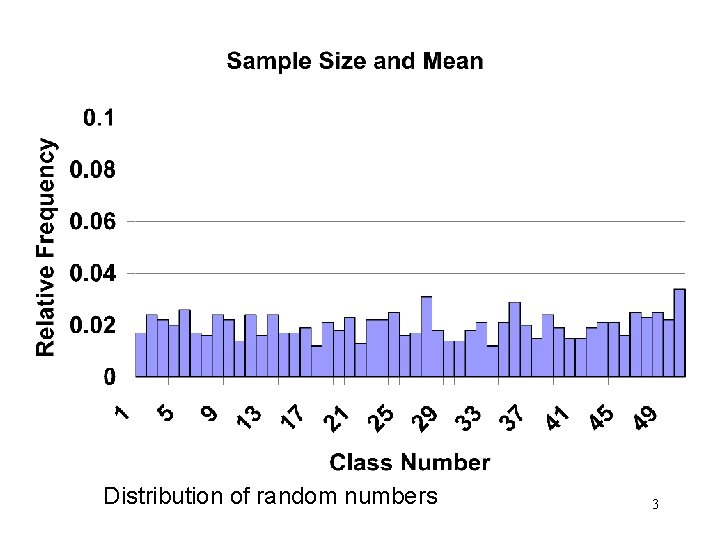Distribution of random numbers 3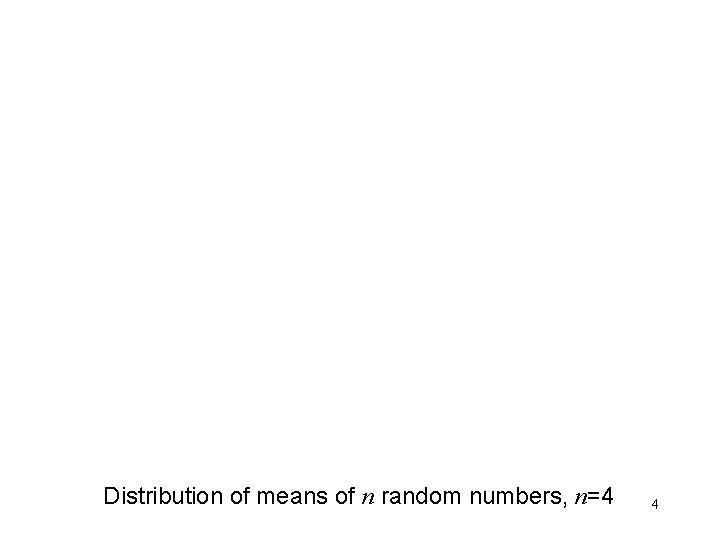Distribution of means of n random numbers, n=4 4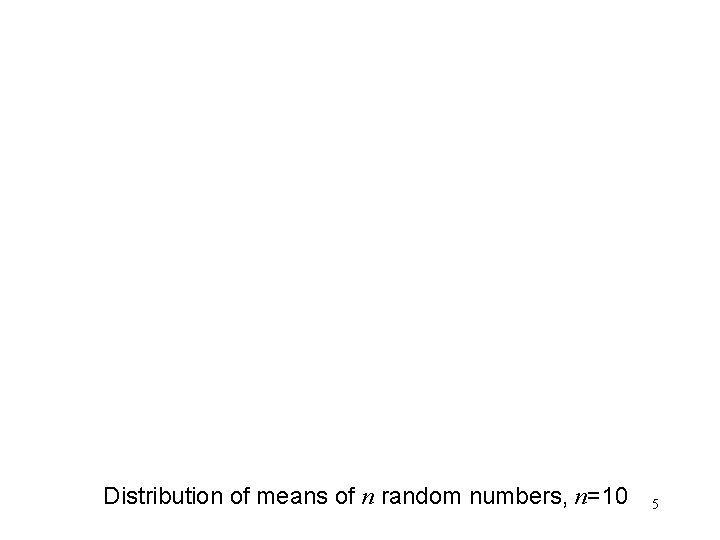Distribution of means of n random numbers, n=10 5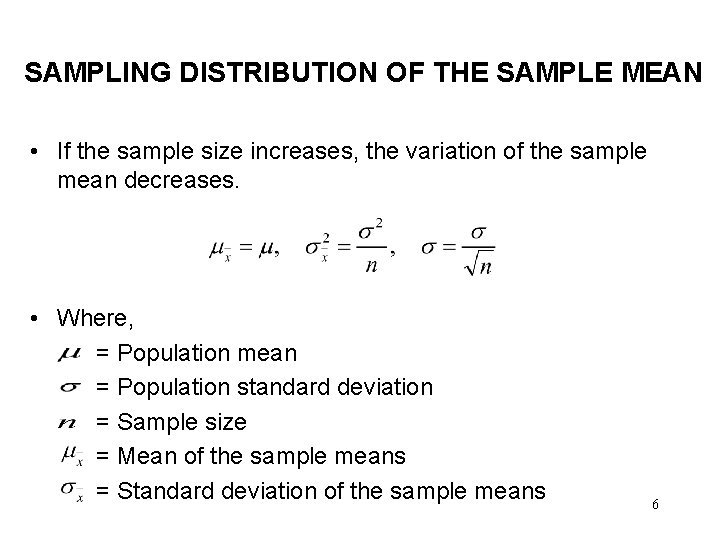SAMPLING DISTRIBUTION OF THE SAMPLE MEAN • If the sample size increases, the variation of the sample mean decreases. • Where, = Population mean = Population standard deviation = Sample size = Mean of the sample means = Standard deviation of the sample means 6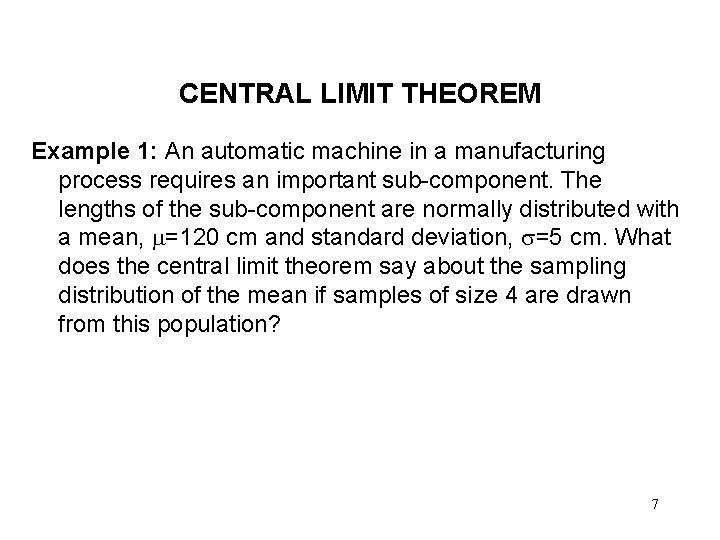CENTRAL LIMIT THEOREM Example 1: An automatic machine in a manufacturing process requires an important sub-component. The lengths of the sub-component are normally distributed with a mean, =120 cm and standard deviation, =5 cm. What does the central limit theorem say about the sampling distribution of the mean if samples of size 4 are drawn from this population? 7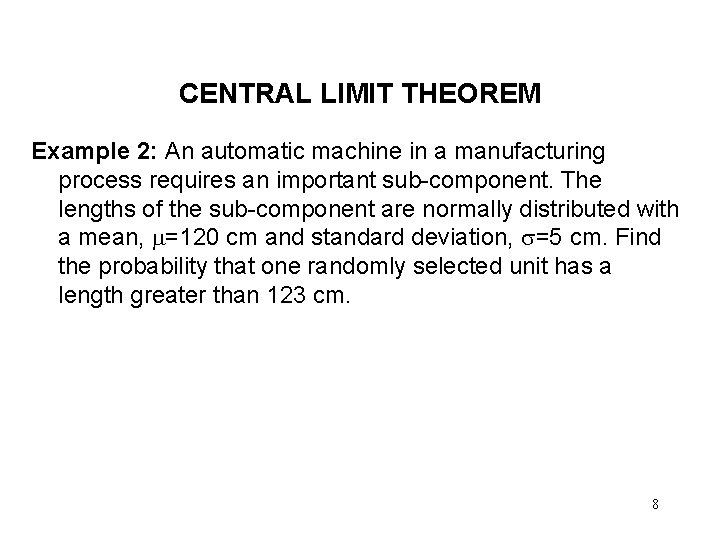CENTRAL LIMIT THEOREM Example 2: An automatic machine in a manufacturing process requires an important sub-component. The lengths of the sub-component are normally distributed with a mean, =120 cm and standard deviation, =5 cm. Find the probability that one randomly selected unit has a length greater than 123 cm. 8CENTRAL LIMIT THEOREM Example 3: An automatic machine in a manufacturing process requires an important sub-component. The lengths of the sub-component are normally distributed with a mean, =120 cm and standard deviation, =5 cm. Find the probability that, if four units are randomly selected, their mean length exceeds 123 cm. 9CENTRAL LIMIT THEOREM Example 4: An automatic machine in a manufacturing process requires an important sub-component. The lengths of the sub-component are normally distributed with a mean, =120 cm and standard deviation, =5 cm. Find the probability that, if four units are randomly selected, all four have lengths that exceed 123 cm. 10READING AND EXERCISES • Reading: pp. 289 -298 • Exercises: 8. 2, 8. 4, 8. 6 11